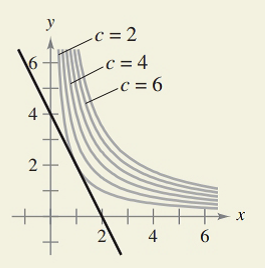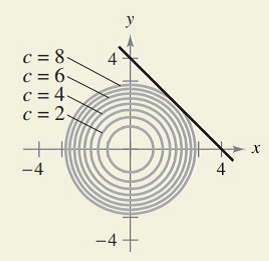Chapter 7.6, Problem 44E### Calculus: An Applied Approach (Min...

10th Edition
Ron Larson
ISBN: 9781305860919

#### Solutions

Chapter
Section### Calculus: An Applied Approach (Min...

10th Edition
Ron Larson
ISBN: 9781305860919
Textbook Problem
567 views

# HOW DO YOU SEE IT? The graphs show the constraint and several level curves of the objective function. Use the graph to approximate the indicated extrema.(a) Maximize z = xyConstraint: 2 x + y =   4(b) Minimize z   =   x 2 + y 2 Constraint: x + y  -  4   =   0a)

To determine

To calculate: The maximum value of objective function z=xy subject to the constraint, 2x+y= 4

Explanation

Given Information:

The provided objective function is z=xy subject to the constraint, 2x+y= 4

Formula used:

If f(x,y) has a maximum or a minimum subjected to constraint g(x,y)=0, then it will occur at one of the critical points defined by function F as

F(x,y,λ)=f(x,y)λg(x,y)

This method is the of Lagrange multipliers where λ is a Lagrange multiplier.

The following equations are to be solved,

Fx(x,y,λ)=0Fy(x,y,λ)=0Fλ(x,y,λ)=0

f(x,y) is evaluated at every point as obtained above:

Calculation:

Let f(x,y)=xy and g(x,y)=2x+y4, then define a new function as:

F(x,y,λ)=f(x,y)λg(x,y)=xyλ(2x+y4)

Now to find the critical numbers of F, find the partial derivative of F with respect to x,y,λ and put them equal to zero

b)

To determine

To calculate: The maximum value of objective function z=x2+y2 subject to the constraint, x+y4=0.

### Still sussing out bartleby?

Check out a sample textbook solution.

See a sample solution

#### The Solution to Your Study Problems

Bartleby provides explanations to thousands of textbook problems written by our experts, many with advanced degrees!

Get Started

#### In Exercises 107-120, factor each expression completely. 119. x6 + 125

Applied Calculus for the Managerial, Life, and Social Sciences: A Brief Approach

#### In Problems 19-44, factor completely. 27.

Mathematical Applications for the Management, Life, and Social Sciences

#### What is the partial fraction form of ?

Study Guide for Stewart's Single Variable Calculus: Early Transcendentals, 8th